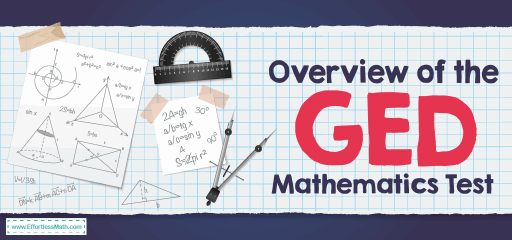# Overview of the GED Mathematical Reasoning TestThe General Educational Development test, or General Education Diploma, commonly known as the GED is a secondary option for people who did not complete high school and receive their high school diploma. The GED, recognized in all 50 states, acts as a high school equivalency credential. With this certificate, graduates can apply for colleges and early-level employment opportunities.

The GED Mathematical Reasoning test is one of the four-course tests that you must pass to get your GED. The GED Mathematical Reasoning Test measures quantitative and algebraic problem-solving skills and requires a test-taker to demonstrate an understanding of math concepts, the skill to use these concepts, and the ability to apply knowledge in real-world scenarios.

The test consists of four skill areas: Quantitative problem-solving in measurement (20%), Quantitative problem-solving with rational numbers (25%), Algebraic problem-solving with expressions and equations (30%), and Algebraic problem solving with graphs and functions (25%).

## How is the GED Mathematical Reasoning test structured?

Like all GED tests, the GED Mathematical Reasoning test is a computer-based test that must be taken at a formal test center. This test has two parts: the first part has five questions in which the use of a calculator usage is prohibited, the second part contains 41 questions for which you may use a calculator. The sections are not timed individually, you have 115 minutes (1 hour and 55 minutes) to complete the entire test. When taking the test, you can use an on-screen calculator or a TI-30XS scientific calculator.

On the GED Math test, you will encounter 6 types of questions, including:

### 1- Multiple-choice:

This is the most common type of assessment. Multiple choice questions ask the student to choose one correct answer from 4 or 5 possible answer options.

### 2- Multiple-select:

These types of questions are slightly different from multiple-choice. Multiple-select questions ask the students to choose all the correct answer options from several choices. Instead of just one correct answer, you can have two or more correct answers.

### 3- Fill-in-the-blank:

These types of questions ask the student to type their answer in a box after the question, or a box that is part of the sentence. In mathematics, the answer is often numerical but can be a written word or a short phrase.

### 4- Drag-and-drop:

This type of question has draggable answer options. When the student clicks and holds on to one of the drag options, he/she can drag it to a “target” area to answer the question correctly. Sometimes there maybe two or more target areas.

### 5- Matching:

This type of question asks the student to mark the box when the information in a column matches the information in a row. For example, one column header could be “true” and another “false”. Students read the information in a row and check whether that information is true or false.

### 6- Table entry:

These types of questions are usually used for a table of values with two columns. Certain cells in the table have a box in which the student types in a number so that the table is correct.

## What type of Math is on the GED Mathematical Reasoning test?

The GED Mathematical Reasoning test includes two types of problems: quantitative problem-solving and algebraic problem-solving. Some of the many topics are:

• Surface area
• Simple interest
• The slope of a line
• Perimeter
• Pythagorean theorem
• Total cost
• Mean & median
• Circumference

## Do you get a formula sheet on the GED Mathematical Reasoning test?

The GED® 2021 Mathematical Reasoning test includes a formula sheet, which shows the formulas for certain algebra concepts and geometric measurements. Formulas are provided to test-takers so that they can focus on the application, instead of the memorization of formulas.

## Is the GED Mathematical Reasoning test hard?

It all depends on how prepared you are. It will not be difficult if you take the time to study for the GED Math test. The hardest part of GED revenue is deciding to do it! Once you’ve done that, the rest is as simple as following this guide and spending a little time and effort.

## How is the GED Mathematical Reasoning test scored?

The GED scoring system is complex, so a question is not always equal to one point. Some questions are filled with blanks or multiple options, where you have to select multiple answers, which means multiple points.

To pass the GED Mathematical Reasoning test, you need at least a score of 145. Passing scores are divided into three categories, indicating whether you have demonstrated skills equal to a high school graduate, a person starting at college, or a college student who has taken undergraduate math courses. The last designation may make you eligible for as many as 3 math courses at some universities and colleges.

## More from Effortless Math for GED Test …

Now, you have learned everything you need to know about the GED Math test. If you are looking for a free GED Math course check out our Ultimate GED Math Course. It contains lessons, videos, worksheets, practice tests, and everything a test taker needs to ace the GED Math test.

### Not sure which GED Math prep book to use?

Take a look at our Favorite Picks for the GED Math at Top 10 GED Math Prep Books.

### Looking for Math worksheets to measure your math skills?

Review our comprehensive GED Math Worksheets to help you practice all GED Math topics and ace the GED Math test.

## Have any questions about the GED Test?

### What people say about "Overview of the GED Mathematical Reasoning Test - Effortless Math: We Help Students Learn to LOVE Mathematics"?

No one replied yet.

X
49% OFF

Limited time only!

Save Over 49%

SAVE \$34

It was \$69.99 now it is \$35.99